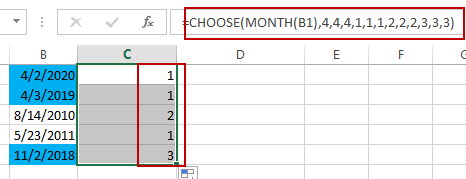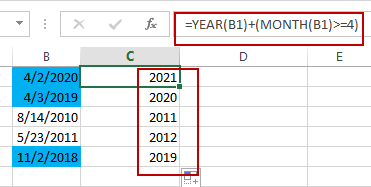# Converting Dates to Fiscal Quarters and Years

This post will guide you how to convert dates to fiscal quarters or years in Excel. How do I get fiscal quarter from a given date in Excel. How to calculate the fiscal year from a date in Excel. How to convert date to fiscal year or month with a formula in Excel.

If  you have a list of data in range B1:B5 which contain dates, and you want to calculate the fiscal quarters and years in your worksheet, how to achieve it. You can refer to the following introduction to achieve the result.

Assuming that the start month of the fiscal year in your company is April.

## Converting Dates to Fiscal Quarters

To calculate one date to a Fiscal quarters in your worksheet, you can use a formula based on the CHOOSE function and the MONTH function. Just like this:

`=CHOOSE(MONTH(B1),4,4,4,1,1,1,2,2,2,3,3,3)`

Select Cell C1, and type this formula into it, and press Enter key in your keyboard, and then drag the AutoFill Handle over other cells to apply this formula.The Fiscal quarters have been calculated.

## Converting Dates to Fiscal Years

To convert dates in cells to Fiscal years, you can create a formula based on the YEAR function and the MONTH function. Just like the following formula:

`=YEAR(B1)+(MONTH(B1)>=4)`

Type this formula in Cell C1, and press Enter key, and then drag the AutoFill Handle over other cells to apply this formula.The Fiscal Years have been converted successfully in your worksheet.

### Related Functions

• Excel Choose Function
The Excel CHOOSE function returns a value from a list of values. The CHOOSE function is a build-in function in Microsoft Excel and it is categorized as a Lookup and Reference Function.The syntax of the CHOOSE function is as below:=CHOOSE (index_num, value1,[value2],…)…
• Excel YEAR function
The Excel YEAR function returns a four-digit year from a given date value, the year is returned as an integer ranging from 1900 to 9999. The syntax of the YEAR function is as below:=YEAR (serial_number)…
• Excel MONTH function
The Excel MONTH function returns the month of a date represented by a serial number. And the month is an integer number from 1 to 12. The syntax of the MONTH function is as below:=MONTH (serial_numbe…